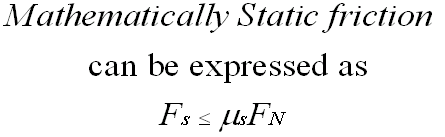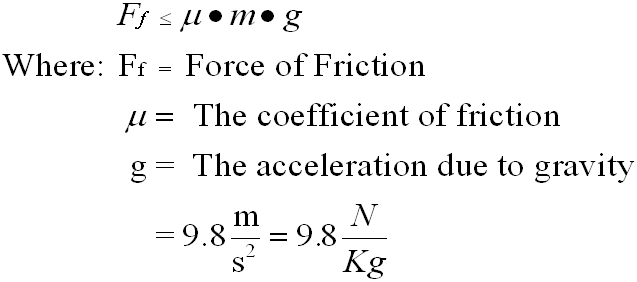### The force of Friction

Friction is generally defined as the "force that opposes" motion.

THERE ARE TWO TYPES FRICTION FORCES THAT MAY BE ACTING ON THE OBJECT.

When the motion of an object is analyzed, one must take into consideration that

- Static friction:

i) Before the object starts to move

-Kinetic friction

ii) While the object is moving

In our laboratory experiments we also found that friction depends on several factors:

1. The weight of the object i.e. the force of gravity   Where:: Fg = m x g -  Therefore,  we can say that indirectly friction depends on mass

2.  Friction also depends on the type of surface

i.e. Friction was higher on rough surfaces (sand paper vs. plastic)

Surfaces ca be classified according to their degree of roughness.  This indcator is called  the Coefficient of Friction

The higher the Coefficient of friction, the rougher the surface .: the higher  the friction force.  Therefore:For our purposes we will use the equation##### Note: The force of friction dose not depend on surface Area.

Example:
A 200 Kg wood crate loaded with bricks is pulled along a rubber floor. The coefficient of friction is 0.38. How much force is needed to move the crate?

Given: m = 200 Kg
g = 9.8 m / s2
u = 0.35

Find: Ff

Solution:        Ff =  µ m g

= ( 0.35) (200 Kg) ( 9.8 m / s2)

= 686 Kg m (s2)  =  686 (N)

.: The minimum force required to get the crate moving is 686N